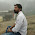# Round Robin Scheduling Program in C++ {Source Code} | Gantt Chart

Implementing a Round Robin Scheduling Algorithm in C++ programming language with a Gantt chart and arrival time. As we all know the Round Robin CPU Scheduling Algorithm, so we have to Write a Program code In C++ language to check how it performs all the operations. We are also going to discuss the Turn around time, burst time and execution time. In this article, you will get all the knowledge about the Round-robin. How it's works and the pro and cons.

## Algorithms Explanation

We can Understand Round Robin Scheduling Algorithm by taking an example Suppose there is 4 process. and each process comes at the same time so based on FIFO(First in First Out) scheduler keep all process in Ready Queue and forgiven time Slice each process will be executed until all process finish. Let's take an example and try to understand How Round Robin Works.

### Round Robin Example

Quantum = 4

Now Schedular keeps all process in Ready Queue and based on FIFO(First in First Out) send the first process for execution.

P1    P2    P3    P4    P1    P3    P4    P4

0      4      7      11     12    16    20     21

Average waiting time = 10.75
Average turn around time = 17

### What is Round Robin Scheduling Algorithm?.

Round Robin is a primitive Scheduling Algorithm and most important and commonly used scheduling algorithm for CPU. In Round Robin Scheduling Algorithm each process has its own execution time that is called "Quantum".

After Quantum time next process start executes for given Quantum time and so on once a cycle complete again process execution start from first, process and repeat the process again and again and for saving a current state of process Context switching is used.

• Round Robin is a primitive Scheduling Algorithm.
• Round Robin follow FIFO(First in First Out) Principle.
• For executing each process in Round Robin Time cluster or time Slice provides, so a process can execute for a particularly given amount of time, the given time is called Quantum.
• After Quantum time for saving a state of each process Context switching is used.
• Round Robin is Great to use for fully Utilization of a CPU and Multitasking.
• Scheduler always needs to keep ready next process ready in ready Queue or Queue for execution in CPU so we can say that scheduler play an important role in the round-robin.
• After Quantum Time for each process, the same step repeats again and again.

### How to Calculate Turn Around Time?.

Turn Around Time = Completion Time – Arrival Time, With the help of this formula, we can calculate a Turn Around Time of all process in Queue.

### How to Calculate Waiting Time?.

Waiting Time = Turn Around Time – Burst Time, This formula is used for calculating the waiting time for the rest of the process.

## Round Robin Program in C++ Language

#include<iostream>
#include<cstdlib>
#include<queue>
#include<cstdio>
using namespace std;

/* C++ Program to Round Robin*/

typedef struct process
{
int id,at,bt,st,ft,pr;
float wt,tat;
}process;

process p,p1,temp;
queue<int> q1;

int accept(int ch);
void turnwait(int n);
void display(int n);
void ganttrr(int n);

int main()
{
int i,n,ts,ch,j,x;

p.tat=0;
p.wt=0;

n=accept(ch);
ganttrr(n);
turnwait(n);

display(n);

return 0;
}

int accept(int ch)
{
int i,n;

printf("Enter the Total Number of Process: ");
scanf("%d",&n);

if(n==0)
{
printf("Invalid");
exit(1);
}

cout<<endl;

for(i=1;i<=n;i++)
{
printf("Enter an Arrival Time of the Process P%d: ",i);
scanf("%d",&p[i].at);
p[i].id=i;
}

cout<<endl;

for(i=1;i<=n;i++)
{
printf("Enter a Burst Time of the Process P%d: ",i);
scanf("%d",&p[i].bt);

for(i=1;i<=n;i++)
{
p1[i]=p[i];
}
return n;
}

void ganttrr(int n)
int i,ts,m,nextval,nextarr;

nextval=p1.at;
i=1;

cout<<"\nEnter the Time Slice or Quantum: ";
cin>>ts;

for(i=1;i<=n && p1[i].at<=nextval;i++)
{
q1.push(p1[i].id);
}

while(!q1.empty())

m=q1.front();
q1.pop();

if(p1[m].bt>=ts)
{
nextval=nextval+ts;
else
{
nextval=nextval+p1[m].bt;
}
if(p1[m].bt>=ts)
{
p1[m].bt=p1[m].bt-ts;
else
{
p1[m].bt=0;
}

while(i<=n&&p1[i].at<=nextval)
{
q1.push(p1[i].id);
i++;
}

if(p1[m].bt>0)
{
q1.push(m);
}
if(p1[m].bt<=0)
{
p[m].ft=nextval;
}
}
}

void turnwait(int n)
{
int i;

for(i=1;i<=n;i++)
{
p[i].tat=p[i].ft-p[i].at;
p[i].wt=p[i].tat-p[i].bt;
p.tat=p.tat+p[i].tat;
p.wt=p.wt+p[i].wt;
}

p.tat=p.tat/n;
p.wt=p.wt/n;
}

void display(int n)
{
int i;

/*
Here
at = Arrival time,
bt = Burst time,
time_quantum= Quantum time
tat = Turn around time,
wt = Waiting time
*/

cout<<"\n=====================================================\n";
cout<<"\n\nHere AT = Arrival Time\nBT = Burst Time\nTAT = Turn Around Time\nWT = Waiting Time\n";

cout<<"\n===================TABLE==============================\n";
printf("\nProcess\tAT\tBT\tFT\tTAT\t\tWT");

for(i=1;i<=n;i++)
{
printf("\nP%d\t%d\t%d\t%d\t%f\t%f",p[i].id,p[i].at,p[i].bt,p[i].ft,p[i].tat,p[i].wt);
}
cout<<"\n=====================================================\n";
printf("\nAverage Turn Around Time: %f",p.tat);
printf("\nAverage Waiting Time: %f\n",p.wt);
}

### The Output of Scheduling Code

#### Pro

• One of the oldest, simple, commonly used scheduling algorithms

#### Cons

• Do not consider priority

#### What is Arrival Time?

Ans- The time at which the process enters into the ready queue.

#### What is Turnaround Time?

Ans- The interval between the times of submission of a process to the time of completion.

#### What is Waiting Time?

Ans- The total amount of the time a process spends in the ready queue.

#### What is Burst Time?

Ans- The time needed by CPU to complete its execution.

#### What is Quantum time?

Ans- The amount of the time a CPU is assigned to be executed is known as the quantum time independent of the actual burst time, a process will get scheduled in quantum parts values or we can say in quantum chunks.

Practice more with Given Example.

### Related Articles

1.1.